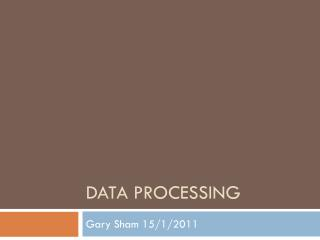DownloadDownload PresentationDATA PROCESSING

# DATA PROCESSING

Télécharger la présentation## DATA PROCESSING

- - - - - - - - - - - - - - - - - - - - - - - - - - - E N D - - - - - - - - - - - - - - - - - - - - - - - - - - -
##### Presentation Transcript

1. DATA PROCESSING Gary Sham 15/1/2011

2. Data Processing • Data Processing ≠ Algorithm • Data Processing: • Get the input so that we can use it in our program. • Save the input by some method to make it easier to code. • How to prevent WA. • Our Goal: A general method to do Data Processing. • Practice makes perfect. • It is always useful in all programming problems. Input Processing Output

3. Data Types The table is by no means complete and the correspondence is not exact. www.google.com

4. Floating Point • Floating Point Representation leads to Precision Error • 1/3  0.3333333 • 0.15  0.149999 • Always try to use ordinal types instead, e.g. Integer. • More accurate; and • Operate faster generally. • Use most accurate floating type if possible. • Use some special operation to handle it.

5. Conversion to integer • How to handle floating point data? • Convert them to integers if possible • Integers are easy to manipulate • E.g. Given numbers to 2 decimal places • 123.45  12345 • 0.10  10 • a := round(d*100); • a = (int)(d*100 + 0.5) • Scanf is useful… • scanf(“%d.%d”, &a,&b);

6. Special operations • Output : • writeln((a+1e-10):0:2) • printf(“%.2lf\n”,a+1e-10); • Compare: • a==b • abs(a-b) < 1e-8 • a < b • a < b – 1e-8 • a <= b • a < b + 1e-8 • ……

7. String • Pascal: • 1 byte storing length and 255 bytes storing array of characters. • Total size is 256 bytes by default. • C/C++ • Null-terminating array of characters. Last byte (Null-character) denotes the end.

8. String processing • Pascal: normal assignment • s := ’abcde’; • t := s; • s := ’9’; • C: by char *strcpy(char *dest, const char *src); • strcpy(s, "abcde"); • strcpy(t, s); • s = ’9’; • C++: normal assignment • s = "abcde"; • t = s; • s = ’9’; • Google it……

9. Hints • Do not use C function strlen in a loop…… • e.g. for (int i=0;i<strlen(s);++i) {…} • C string is Null-terminating. • Also be careful on all string operations(strcpy, etc.) • int n = strlen(s); for (int i=0;i<n;++i) {…}

10. Packed Data Types • Record/struct • It increase the readability. • It is important when debugging. • Operator overloading(C++)

11. Mapping • Swapping the data may be slow • Records in Pascal • Strings/Struct in C/C++ • Arrays • Use a pointer to represent the data.

12. Hard-coding • Hard-coding is important technique in tackling data processing problems • 2067 – Tappy Game • Blocks MUST be hard-coded • What should we hard-code? • Number of blocks occupied for each column of each Tappy • 4 rotations of each Tappy • Hard-coding is much easier than writing codes for rotation!

13. Hard-coding • When you are doing some searching in a maze. • DFS/BFS? • Yes, but HOW? • if (x+1>0) && (x+1<n) && (y>0) && (y<n){…} • if (x-1>0) && (x-1<n) && (y>0) && (y<n){…} • if (x>0) && (x<n) && (y+1>0) && (y+1<n){…} • if (x>0) && (x<n) && (y-1>0) && (y-1<n){…} • ……………………

14. Hard-coding • How about 8 directions?! • int dy={1,1,-1,-1,0,0,0,0}; • int dx={0,0,0,0,1,1,-1,-1}; • We can use for loop now! :D • Shorter code

15. Exercise • 1012 Allocating School Places • 2042 Reversi • 2030 Be Jewelled! • 2080 Simple Calculator • 2067 Tappy Game